# Qlik Design Blog

All about product and Qlik solutions: scripting, data modeling, visual design, extensions, best practices, etc.

Announcements
Join “Do More With Qlik” with Mike Tarallo to get a first-hand look at the New Authoring Experience, June 29th: REGISTER HEREEmployee

## Regression Lines

What is a regression line? In simple terms, it is a line that best describes the behavior of a set of data. They are often used for forecasting to illustrate the relationship between the dependent y variable and the independent x variables when there is linear pattern. Using a regression line can show future behavior of the dependent variable using different inputs for the independent x variables. Scatter plots now support regression lines and provide many options to choose from. In the properties pane of the scatter plot chart under Add-ons, is an option for Regression Lines.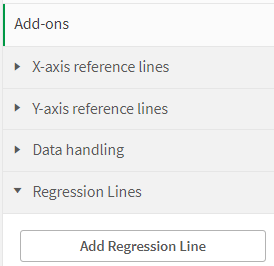Click the Add Regression Line button to add a regression line to your chart. There are many types to choose from depending on your data and what you would like to show. According the Qlik Help, here are the regression line types available to add to a scatter plot chart.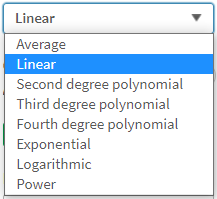• Average: Shows the average value of the data.
• Linear: Shows a linear increase or decrease of values.
• Second Degree Polynomial: Shows a curved line to represent fluctuating data with one hill or valley.
• Third Degree Polynomial: Shows a curved line to represent fluctuating data with up to two hills or valleys.
• Fourth Degree Polynomial: Shows a curved line to represent fluctuating data with up to three hills or valleys.
• Exponential: Shows a curved line. Use when data values rise or fall at increasingly higher rates.
• Logarithmic: Shows a curved line. Use when the rate of change in data increases or decreases quickly, then levels out.
• Power: Shows a curved line. Use with data sets that compare measurements that increase at specific rates.

Here are some regression line examples: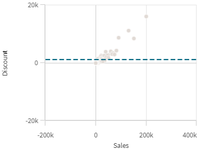Average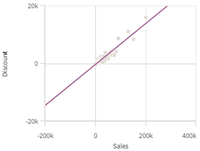LinearSecond Degree Polynomial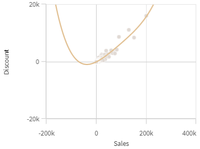Fourth Degree Polynomial

There are some additional properties that can be set for a regression line. The color of the line and label can be set, and the line can be displayed as solid or dashed. The direction of fit can also be set to either minimize vertically or minimize horizontally. A scatter plot can also have more than one regression as seen in the chart below.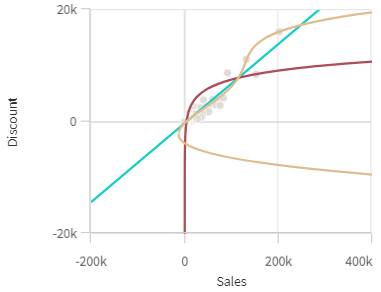Regression lines are helpful for analysis because it allows us to see patterns in our data. They allow us to see the relationships between two or more variables and examine which variables may have an impact. Check them out - Qlik Sense makes it easy to use them in your scatter plots charts.

Thanks,

Jennell